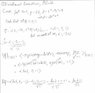# Directional Derivative

jegues

## Homework Statement

Find the rate of change of the function,

$$f(x,y,z) = cos(\pi x y) + xln(z^{2}+1),$$

with respect to length $$s$$ along the curve,

$$y=-3x, z=x^{2}-y^{2}+9,$$

directed so that x increases, at the point (-1,3,1).

## The Attempt at a Solution

See figure attached.

I wrote parametric equations for the curve, and threw them into a vector.

Since at the point (-1,3,1) t=-1, I evaluated the vector accordingly.

The question says "so that x increase" so I reversed the direction of the vector by multiplying it by negative 1.

After this I found a unit vector that points in the same direction as the vector described previously.

Then I found the gradient of f and evaluated it at the point (-1,3,1).

After this I dotted the two to get the rate of change of f in the desired direction.

The solution lists the answer as,

$$\frac{ln(2) - 16}{\sqrt{266}}$$

Where did I go wrong?

#### Attachments

•SEQ3.jpg
24.2 KB · Views: 325